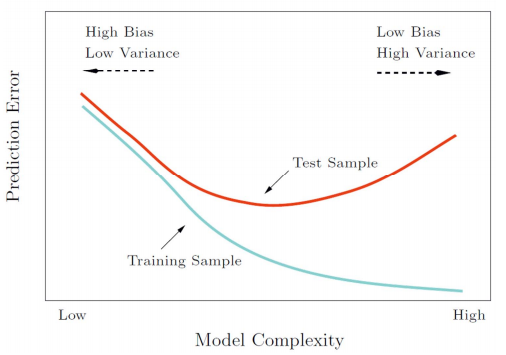# Data Mining

## Intro

• Good representation:
• Low intra‐class variability
• Low inter‐class similarity
• Good representation: Simple model (classifier)
more important in real application
• Histograms
• Probability Densities
• Bias and Variance• Generalization of the classifier (model)
The performance of the classifier on test data

## Bayesian

• Bayes' Theorem:
$$P(A|B) = \frac{P(B\mid A)P(A)}{P(B)}$$

• Decision rule with only the prior information
Decide $\omega_1$ if $P(\omega_1)\gt P(\omega_2)$, otherwise decide $\omega_2$

• Likelihood
$P(x\mid \omega_j)$is called the likelihood of $\omega_j$ with respect to $x$; the category $\omega_j$ for which $P(x\mid \omega_j)$ is large is more likely to be the true category

• Maximum likelihood decision
Assign input pattern $x$ to class $\omega_1$ if $P(x \mid \omega_1) > P(x \mid \omega_2),$ otherwise $\omega_2$

• Posterior
$$P(\omega_i\mid x)=\frac{P(x\mid \omega_i)P(\omega_i)}{P(x)}\\ P(x)=\sum_{i=1}^{k}{P(x\mid \omega_i)P(\omega_i)}$$
Posterior = (Likelihood × Prior) / Evidence

• Optimal Bayes Decision Rule
Decision given the posterior probabilities, Optimal Bayes Decision rule
Assign input pattern $x$ to class $\omega_1$ if $P(\omega_1 \mid x) > P(\omega_2 \mid x),$ otherwise $\omega_2$
Bayes decision rule minimizes the probability of error, that is the term Optimal comes from.
$$P(error\mid x) = \min{\left\{P(\omega_1\mid x),P(\omega_2\mid x)\right\}}$$

• Special cases:

1. if $P(\omega_1)=P(\omega_2)$, decide $\omega_1$ if $P(x\mid \omega_1)\gt P(x\mid \omega_2)$, otherwise $\omega_2$. (MLD)
2. if $P(x\mid \omega_1) = P(x\mid \omega_2)$, decide $\omega_1$ if $P(\omega_1)\gt P(\omega_2)$, otherwise $\omega_2$.
• Bayes Risk

• Conditional risk
$\alpha_i$ is action and $\lambda(\alpha_i\mid \omega_i)$ is he loss incurred for taking action $\omega_i$ when the true state of nature is $\omega_j$.
$$R(\alpha_i \mid \boldsymbol{x}) = \sum_{j=1}^{c}{\lambda(\alpha_i\mid \omega_j)P(\omega_j\mid \boldsymbol{x})}$$
• Bayes Risk
$$R=\sum_{\boldsymbol{x}}{R(\alpha_i\mid \boldsymbol{x})}$$
• Minimum Bayes risk = best performance that can be achieved
• Likelihood Ratio
if
$$\frac{P(\boldsymbol{x}\mid\omega_1)}{P(\boldsymbol{x}\mid\omega_2)}\gt \frac{\lambda_{12}-\lambda_{22}}{\lambda_{12}-\lambda_{11}} \times \frac{P(\omega_2)}{P(\omega_1)}$$ decide $\omega_1$.
"If the likelihood ratio exceeds a threshold value that is independent of the input pattern x, we can take optimal actions"

• Minimum Error Rate Classification
$$R(\alpha_x\mid \boldsymbol{x}) = 1-P(\omega_i\mid \boldsymbol{x})$$
Minimizing the risk → Maximizing the posterior $P(\omega_i\mid \boldsymbol{x})$

• Network Representation of a Classifier• Bayes Classifier
$$g_i(\boldsymbol{x}) = P(\boldsymbol{x}\mid \omega_i)P(\omega_i)\\ g_i(\boldsymbol{x}) = \ln{P(\boldsymbol{x}\mid \omega_i)}+\ln{P(\omega_i)}$$

• Binary classification → Multi‐class classification

• One vs. One
• One vs. Rest
• ECOC
• Normal Distribution
$\cal{N}(\boldsymbol{\mu}, \Sigma)$(with dimension $d$)
$$P(\boldsymbol{x})= \frac{1}{\left(2\pi\right)^{\frac{d}{2}}\left|\Sigma\right|^{\frac{1}{2}}}{\exp{\left[-\frac{1}{2}\left(\boldsymbol{x}-\boldsymbol{\mu}\right)^T\Sigma^{-1}\left(\boldsymbol{x}-\boldsymbol{\mu}\right)\right]}}$$

• Maximum‐Likelihood Estimation

$$\nabla_{\theta}=\left[\frac{\partial}{\partial\theta_1},\cdots,\frac{\theta}{\partial\theta_p}\right]$$
• Optimal Estimation
$$\nabla_\theta l=\sum_{k=1}^{n}\ln{P(x_k\mid\theta)}=0$$
• Gaussian with unknown $\mu$ and $\Sigma$
$$\boldsymbol{\hat{\mu}}=\frac{1}{n}\sum_{k=1}^{n}\boldsymbol{x}_k\\ \boldsymbol{\hat{\Sigma}}=\frac{1}{n}\sum_{k=1}^{n}{\left(\boldsymbol{x}_k-\boldsymbol{\hat{\mu}}\right)\left(\boldsymbol{x}_k-\boldsymbol{\hat{\mu}}\right)^T}$$

• Bayesian Estimation
$$P(\omega_i\mid \mathbf{x}, \mathcal{D})=\frac{p(\mathbf{x}\mid \omega_i, \mathcal{D}_i)P(\omega_i)}{\sum_{j=1}^c{p(\mathbf{x}\mid \omega_j, \mathcal{D}_j)P(\omega_j)}}$$
where
$$p(x\mid D)=\int{p(x,\theta\mid D)d\theta}=\int{p(x\mid\theta, D)p(\theta\mid D)d\theta}\\ =\int{p(x\mid \theta)p(\theta\mid D)d\theta}$$
$P(x\mid D)$ is the central problem of Bayesian learning.

• General Theory
$P(x \mid D)$ computation can be applied to any situation in which the unknown density can be parameterized: the basic assumptions are:
• The form of $P(x \mid\theta)$ is assumed known, but the value of $\theta$ is not known exactly
• Our knowledge about $\theta$ is assumed to be contained in a known prior density $P(\theta)$
• The rest of our knowledge about $\theta$ is contained in a set D of n random variables $x_1, x_2, \dots, x_n$ that follows $P(x)$
• Linear Discriminant Analysis
Covariance matrices of all classes are identical but can be arbitrary
$$g_i(x)=\mu_i^T\Sigma^{-1}x-\frac{1}{2}\mu_i^T\Sigma^{-1}\mu_i+\ln{P(\omega_i)}$$

• Estimating Parameters:
• $\mu_i$
$$\mu_i=\frac{1}{N_i}\sum_{j\in\omega_i}x_j$$
• $P(\omega_i)$
$$P(\omega_i)=\frac{N_i}{N}$$
• $\Sigma$
$$\Sigma=\sum_{i=1}^c\sum_{j\in\omega_i}\frac{\left(\boldsymbol{x}_j-\boldsymbol{\mu_i}\right)\left(\boldsymbol{x}_j-\boldsymbol{\mu_i}\right)^T}{N_i}$$
• Error Probabilities and Integrals
$$P(error)=\int_{R_2}{p(x\mid\omega_1)P(\omega_1)dx}+\int_{R_1}{p(x\mid\omega_2)P(\omega_2)dx}$$

• Naive Bayes Classifier
$$P(\omega\mid x_1,\dots,x_p)\propto P(x_1,\dots, x_p\mid \omega)P(\omega)\\ P(x_1,\dots,x_p\mid \omega)=P(x_1\mid \omega)\cdots P(x_p\mid \omega)$$

• Smoothing
$$P(x_i\mid \omega_k)=\frac{\left|x_{ik}\right|+1}{N_{\omega k}+K}$$

## Perception

• Perceptron
$$f(x)=w^Tx+w_0\\ y=sign(f(x))$$
• Learn
• If correct, do nothing
• If wrong, $w_t=w_{t-1}+xy$
• Perceptron Criterion Function
$$E(w)=-\sum_{i\in I_M}w^Tx_iy_i$$
$$x_{n+1}=x_n-\gamma\nabla f(x_n),n\geqslant0$$
Let $J(w)$be the criterion function.
$$w(k+1)=w(k)-\eta(k)\nabla J(w(k))$$
• Batch learning vs. Online learning
• Batch learning
All the training samples are available
• Online learning
The learning algorithm sees the training samples one by one.
• Perceptron Convergence
• converge if the data is linearly separable.
• infinite loop if the training data is not linearly separable

## Linear Regression

• Polynomial Curve Fitting
$$f(x, a)=\sum_{j=0}^M{a_jx^j}\\ MSE(a)=\frac{1}{n}\sum_{i=1}^n{(y_i-f(x_i, a))^2}\\ RSS(a)= \sum_{i=1}^n{(y_i-f(x_i, a))^2}$$
• Linear Regression Model
$$J_n(a)=(y-X^Ta)^T(y-X^Ta)$$
$$a_{k+1}=a_k+\eta_k2X(X^Ta-y)$$
$$\hat{y}=X^Ta=X^T(XX^T)^{-1}Xy$$
• A generative model: $y=f(x,a)+\epsilon$, where $\epsilon\sim N(0,\sigma^2)$. The maximum likelihood estimation result is approximate to RSS.